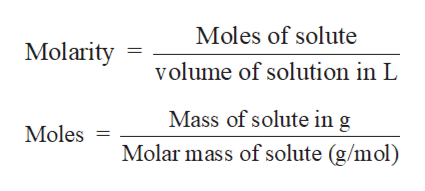# A solution of NaCl(aq) is added slowly to a solution of lead nitrate, Pb(NO3)2(aq), until no further precipitation occurs. The precipitate is collected by filtration, dried, and weighed. A total of 17.07 g PbCl2(s) is obtained from 200.0 mL of the original solution.Calculate the molarity of the Pb(NO3)2(aq) solution.

Question

A solution of NaCl(aq) is added slowly to a solution of lead nitrate, Pb(NO3)2(aq), until no further precipitation occurs. The precipitate is collected by filtration, dried, and weighed. A total of 17.07 g PbCl2(s) is obtained from 200.0 mL of the original solution.

Calculate the molarity of the Pb(NO3)2(aq) solution.

check_circleExpert Solution
Step 1

Solution stoichiometry involves the calculation of concentration of solutions in the given conditions of volumes, moles etc.

Step 2

Solution stoichiometry is mainly based on the calculation of moles and volumes. These two values are used to calculate the molarity of solution. The relation between moles, volume and molarity is as given below;help_outlineImage TranscriptioncloseMoles of solute Molarity volume of solution in L Mass of solute in g Molar mass of solute (g/mol) Moles fullscreen
Step 3

The balance chemical reaction of Pb(NO3)2 with...

### Want to see the full answer?

See Solution

#### Want to see this answer and more?

Solutions are written by subject experts who are available 24/7. Questions are typically answered within 1 hour*

See Solution
*Response times may vary by subject and question
Tagged in

### Solutions